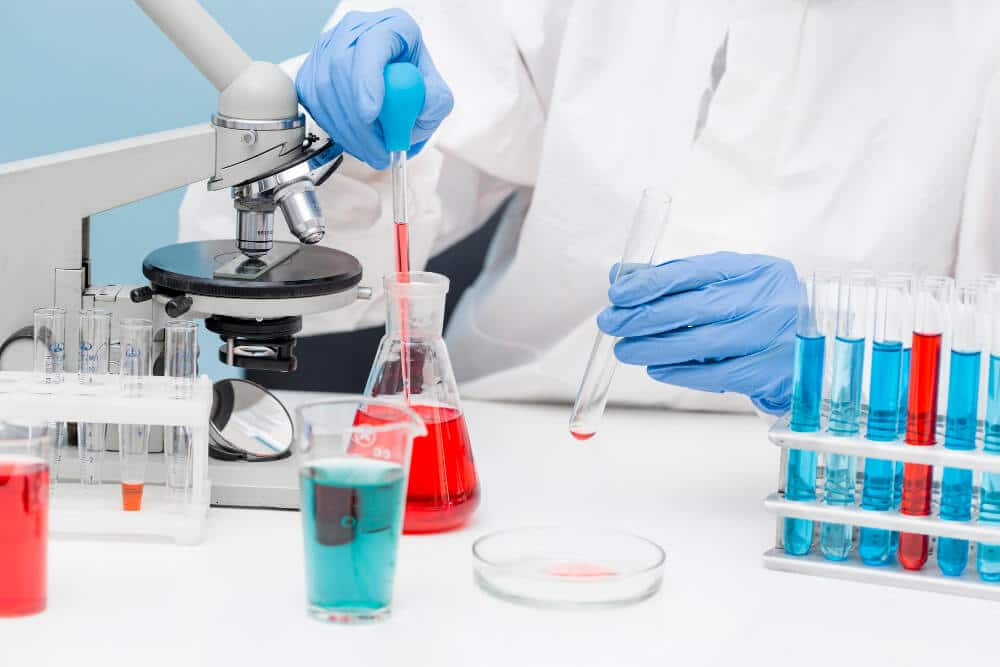Select Page

# Calculate the Stoichiometry Problems Easily and Finding the Numbers of Product Molecules Formed in a ReactionBy Sarah J Mitchell
30 Mar, 2018
K
L

The chemistry of stoichiometry or in other words, the chemistry for massive creatures describes everything one can see around. It helps to understand the biggest concepts of universe by helping us understand the tiniest.
Chemistry traffics in infinitesimal particles and deals with quadrillions of those present around. Stoichiometry is basically thee study or the calculation of the relationship between the different molecules in a reaction. It includes both the reactants or reagents and products taking part in a balanced chemical equation.
It is conceptually quantitative as well as qualitative and records the yield of the product formed during the reaction.

• For most types of chemical reactions there are basically three types of conversion to consider.

The first type is to convert the moles of a substance A to thee moles of substance B.
Here one needs to identify the molar ratio in order to find the solution.

• The second type is to convert the moles of substance A to grams of substance of B.

Simultaneously one also needs to convert the grams of substance of A into moles of substance B.

• The third type is when one is given the grams of substance A that it needed to convert to grams of substance B.

The first type is a single step process while the second and third one is binary and multi step process respectively.
To apply the concept into problem we need to perform sum numerical and see how this works in the practical environment.
Taking a sample problem,

1. Q) After complete reaction, how many molecules of ammonia are produced? If the given amount of hydrogen is 7.o g.

Writing its equation at first, we have
N2 + 3H2 Ã  2NH3
To determine the moles of ammonia formed from the given moles of hydrogen, we first need a conversion factor to go from the moles of hydrogen to moles of ammonia. Here we need a mole ratio for the conversion; that is for every 3 moles of hydrogen in the balanced equation, we make 2 moles of ammonia.
Simply put, 3 mole of H2 = 2 mole of NH3
Hence, solving the equation we find the answer is 4.67 mol of NH3
Therefore we get 7 moles of hydrogen makes 4.67 mol of ammonia.
Proceeding to the next problem,

1. Q) After complete reaction, how many molecules of ammonia are produced? Here given amount of nitrogen is 2g.

In this case, we need to find the grams of ammonia prepared from two moles of nitrogen. Here, the mole ratio and two conversion factors and molar mass of ammonia.
We know as per the balanced equation, 17. O g NH3 = 1 mole NH3
And, 1 g N2 = 2 moles NH3
After calculating, the answer is 68.0 g.
Stoichiometry is all about the calculation of the reactants and products as well as the reagents taking part in a chemical reaction. It also helps to determine the relationship between each. Hence the concept is both crucial as well as complex to understand and holds great weightage in the analytical field of chemistry.